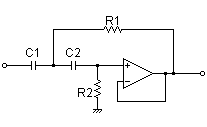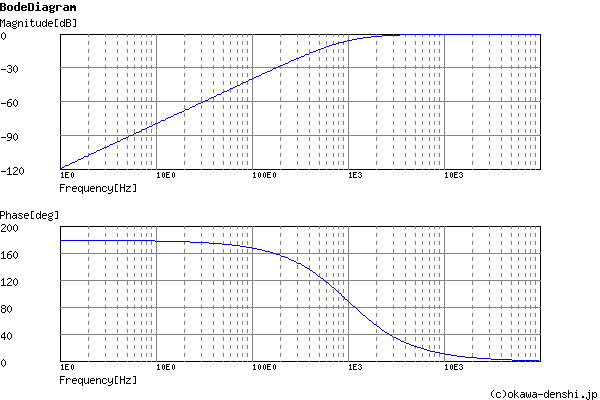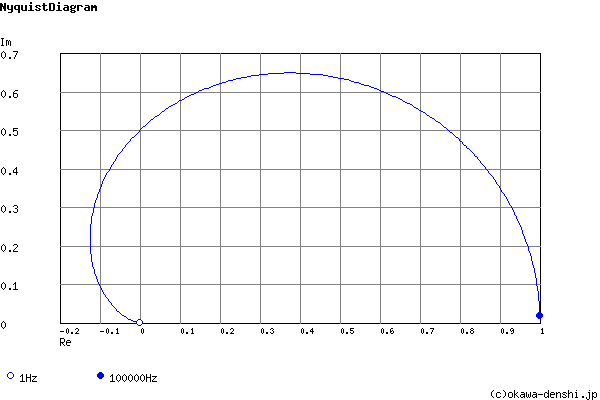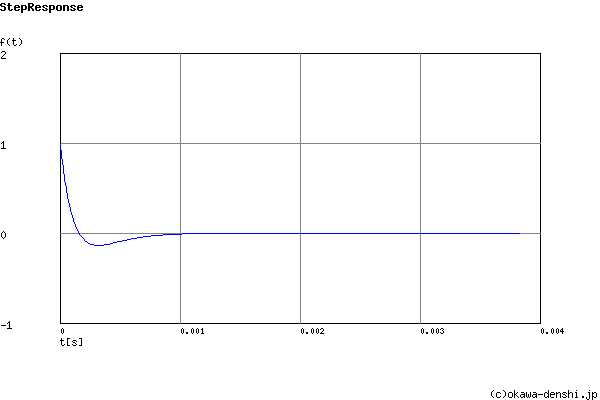# (Sample)Sallen-Key High-pass Filter Design Tool - Result -

Calculated the Transfer Function for the Sallen-Key high-pass filter, displayed on graphs, showing Bode diagram, Nyquist diagram, Impulse response and Step response.

## Sallen-Key High-pass Filter

 Vin(s)→→Vout(s)
(Sample)Transfer Function:
 G(s)= s2 s2+12500s+39062500

R1 = 16kΩ
R2 = 16kΩ
C1 = 0.01uF
C2 = 0.01uF

#### Cut-off frequency

fc = 994.718394324[Hz]

Q = 0.5

ζ = 1

#### Pole(s)

p = -994.718394324 +2.59971382044E-06i[Hz]
|p|= 994.718394324[Hz]
p = -994.718394324-6.59896406561E-07i[Hz]
|p|= 994.718394324[Hz]

z = 0[Hz]
|z|= 0[Hz]
z = -0[Hz]
|z|= 0[Hz]

#### Phase margin

pm= NAN[deg] (f =0[Hz])

#### Overshoot (in absolute value)

The 1st peak  gpk = -0.14 (t =0.00033[sec])

g(∞) = 0

fc=Hz

### Q factor | Damping ratio ζ

Quality factor Q =
Damping ratio ζ =
 C1 = F C2 = F
C1, C2 is optional. But when setting these capacitances, C1, C2 of both are needed to give.

Select Capacitor Sequence:
Select Resistor Sequence:

### Frequency analysis

Bode diagram
Phase  Group delay
Nyquist diagram
Pole, zero
Phase margin
Oscillation analysis
Analysis on frequency range:
f1=∼f2=[Hz] (optional)

### Transient analysis

Step response
Impulse response
Overshoot
Final value of the step response
Analysis on time range:
0∼[sec] (optional)

# Frequency analysis# Transient analysis